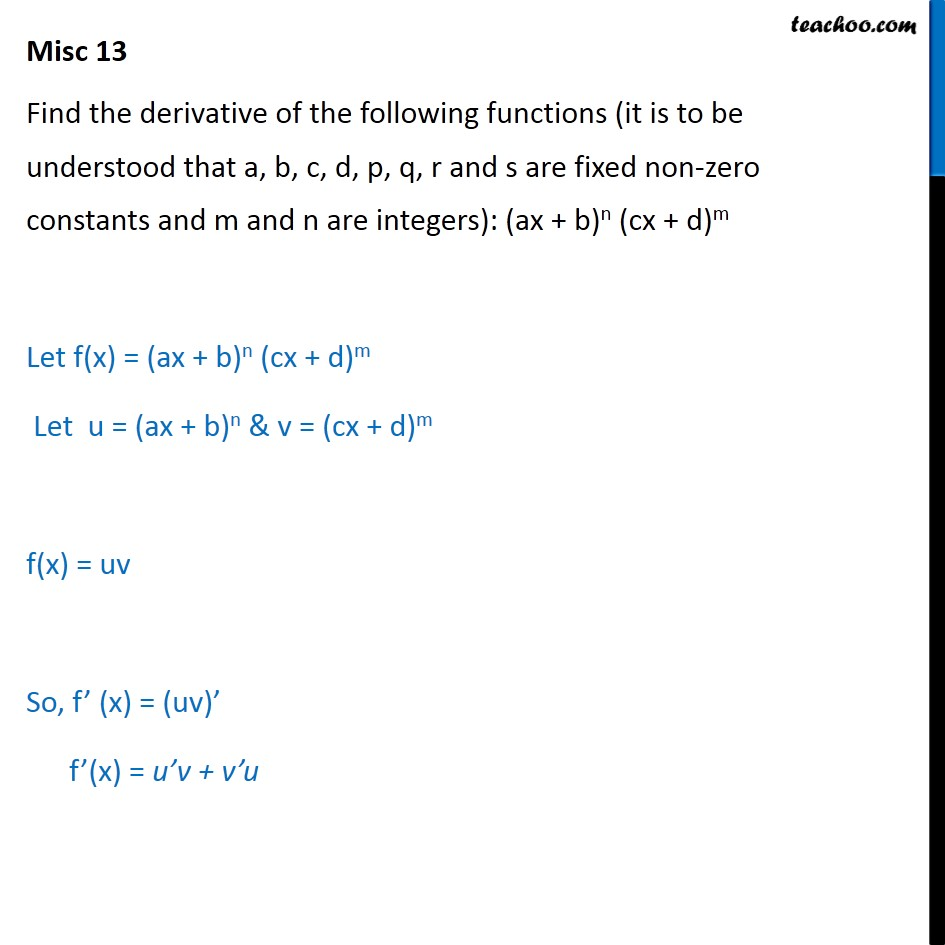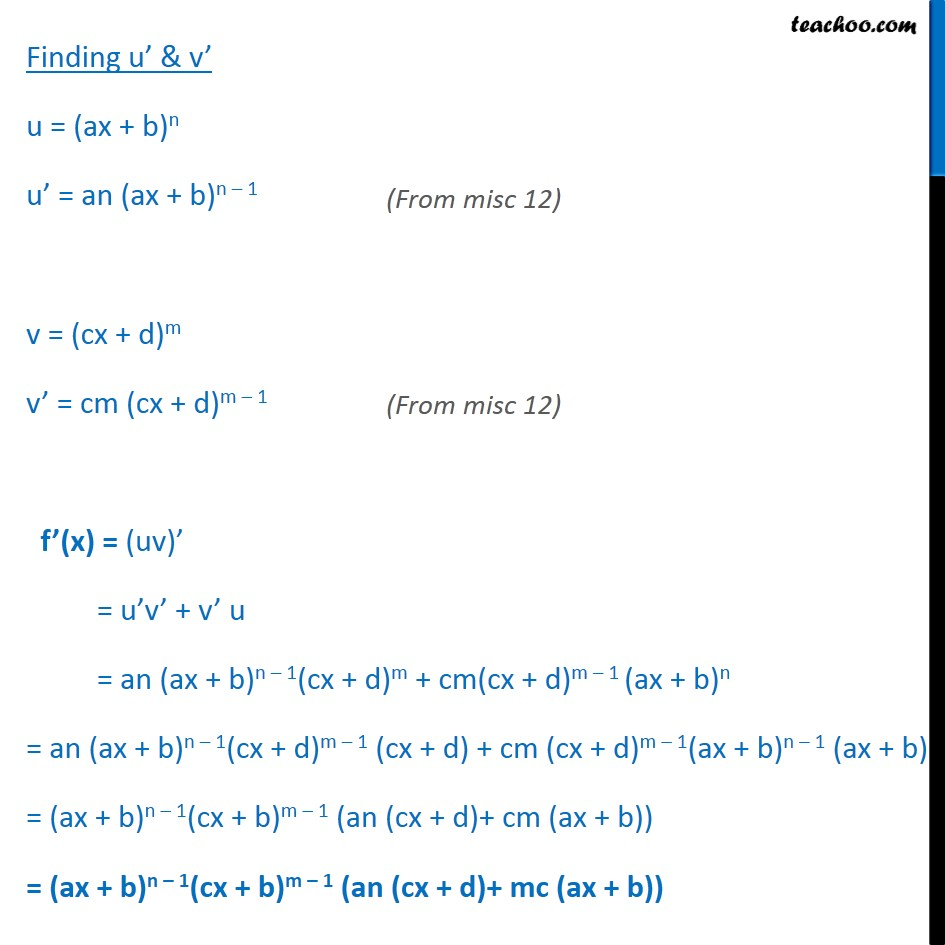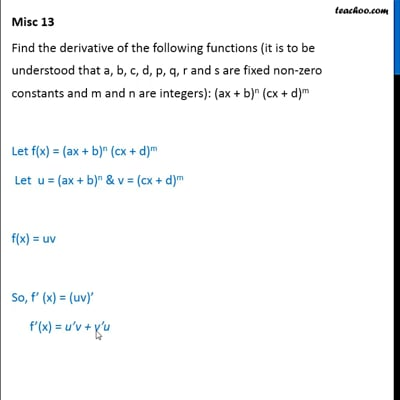Miscellaneous (Term 1 and Term 2)

Chapter 13 Class 11 Limits and Derivatives (Term 1 and Term 2)
Serial order wiseThis video is only available for Teachoo black users

### Transcript

Misc 13 Find the derivative of the following functions (it is to be understood that a, b, c, d, p, q, r and s are fixed non-zero constants and m and n are integers): (ax + b)n (cx + d)m Let f(x) = (ax + b)n (cx + d)m Let u = (ax + b)n & v = (cx + d)m f(x) = uv So, f (x) = (uv) f (x) = u v + v u Finding u & v u = (ax + b)n u = an (ax + b)n 1 v = (cx + d)m v = cm (cx + d)m 1 f (x) = (uv) = u v + v u = an (ax + b)n 1(cx + d)m + cm(cx + d)m 1 (ax + b)n = an (ax + b)n 1(cx + d)m 1 (cx + d) + cm (cx + d)m 1(ax + b)n 1 (ax + b) = (ax + b)n 1(cx + b)m 1 (an (cx + d)+ cm (ax + b)) = (ax + b)n 1(cx + b)m 1 (an (cx + d)+ mc (ax + b))